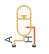# Gas-Charged Accumulator (TL)

Gas-charged accumulator in a thermal liquid network

•Libraries:
Simscape / Fluids / Thermal Liquid / Tanks & Accumulators

## Description

The Gas-Charged Accumulator (TL) block represents a pressurized thermal liquid container with a compressed gas charge. The accumulator consists of thermal liquid and gas chambers separated by a hermetic and insulated diaphragm.

Accumulator SchematicIf the inlet pressure is greater than the gas charge pressure, the liquid chamber volume increases, compressing the gas chamber. If the inlet pressure is lower than the gas charge pressure, the liquid chamber volume decreases, decompressing the gas chamber.

Hard stops limit the diaphragm motion when the liquid chamber is at capacity and when the liquid chamber is empty. If the specified spring stiffness is too low, the liquid volume can momentarily fall below zero or rise above capacity. Use the Hard stop stiffness coefficient parameter to correct this.

### Mass Balance

The mass conservation equation in the liquid chamber is

`${V}_{L}{\rho }_{L}\left(\frac{1}{{\beta }_{L}}\frac{d{p}_{L}}{dt}-{\alpha }_{L}\frac{d{T}_{L}}{dt}\right)+{\rho }_{L}\frac{d{V}_{L}}{dt}={\stackrel{˙}{m}}_{A},$`

where:

• ρL is the thermal liquid density.

• βL is the isothermal bulk modulus.

• αL is the isobaric thermal expansion coefficient.

• pL is the thermal liquid pressure.

• TL is the thermal liquid temperature.

• ${\stackrel{˙}{m}}_{A}$ is the thermal liquid mass flow rate into the accumulator through port A.

The block computes the time variation of the liquid chamber volume using the conditional equation

`$\frac{d{V}_{L}}{dt}=\left\{\begin{array}{ll}\frac{{\stackrel{˙}{p}}_{L}}{k{p}_{pr}{V}_{T}^{k}{V}_{G}^{\left(-k-1\right)}+{K}_{s}+{K}_{d}{\stackrel{˙}{m}}^{+}/{\rho }_{L}},\hfill & {V}_{L}\ge {V}_{C}\hfill \\ \frac{{\stackrel{˙}{p}}_{L}}{k{p}_{pr}{V}_{T}^{k}{V}_{G}^{\left(-k-1\right)}+{K}_{s}-{K}_{d}{\stackrel{˙}{m}}^{-}/{\rho }_{L}},\hfill & {V}_{L}\le 0\hfill \\ \frac{{\stackrel{˙}{p}}_{L}}{k{p}_{pr}{V}_{T}^{k}{V}_{G}^{\left(-k-1\right)}},\hfill & \text{Else}\hfill \end{array},$`

where:

• Ks is the Hard-stop stiffness coefficient parameter.

• Kd is the Hard-stop damping coefficient parameter.

• ${\stackrel{˙}{m}}^{+}$ is the mass flow rate into the liquid chamber when the accumulator diaphragm contacts the top hard stop:

`${\stackrel{˙}{m}}^{+}=\left\{\begin{array}{ll}\stackrel{˙}{m},\hfill & \stackrel{˙}{m}>0\hfill \\ 0,\hfill & \text{Else}\hfill \end{array},$`

• ${\stackrel{˙}{m}}^{-}$ is the mass flow rate from the liquid chamber when the accumulator diaphragm contacts the bottom hard stop:

`${\stackrel{˙}{m}}^{-}=\left\{\begin{array}{ll}\stackrel{˙}{m},\hfill & \stackrel{˙}{m}<0\hfill \\ 0,\hfill & \text{Else}\hfill \end{array},$`

### Momentum Balance

The momentum conservation equation in the accumulator volume is

`${p}_{L}={p}_{G}+{p}_{HS},$`

where pHS is the hard-stop contact pressure:

• `${p}_{HS}=\left\{\begin{array}{ll}\left({V}_{L}-{V}_{C}\right)\left({K}_{s}+{K}_{d}{\stackrel{˙}{m}}^{+}/\rho \right),\hfill & {V}_{L}\ge {V}_{C}\hfill \\ {V}_{L}\left({K}_{s}-{K}_{d}{\stackrel{˙}{m}}^{-}/\rho \right),\hfill & {V}_{L}\le 0\hfill \\ 0,\hfill & \text{Else}\hfill \end{array},$`

### Energy Balance

The energy conservation equation in the liquid chamber volume is

`$\frac{d}{dt}\left({\rho }_{L}{u}_{L}{V}_{L}\right)={\varphi }_{A}+{\varphi }_{H},$`

where:

• uL is the thermal liquid specific internal energy.

• ΦA is the energy flow rate into the liquid chamber through the accumulator inlet.

• ΦH is the thermal energy flow rate into the liquid chamber through the accumulator wall.

### Chamber Volumes

The liquid chamber volume is the difference between the total accumulator volume and the gas chamber volume:

`${V}_{L}={V}_{T}-{V}_{G},$`

where:

• VL is the liquid chamber volume.

• VT is the total accumulator volume.

• VG is the gas chamber volume.

Chamber VolumesThe liquid chamber capacity is the difference between the total accumulator volume and the dead volume of gas in the accumulator at full capacity:

`${V}_{C}={V}_{T}-{V}_{Dead},$`

where:

• VC is the liquid chamber capacity.

The gas chamber pressure and volume follow from the precharge states as described by the polytropic equation

`${p}_{G}{V}_{G}^{k}={p}_{pr}{V}_{T}^{k},$`

where:

• pG is the gas chamber pressure at a given time step.

• VG is the gas chamber volume at a given time step.

• ppr is the precharge gas chamber pressure when the liquid chamber is empty.

• VT is the total liquid chamber volume.

• k is the polytropic index.

### Initial Targets

To set the priority and initial target values for the block variables prior to simulation, use the Initial Targets section in the block dialog box or Property Inspector. For more information, see Set Priority and Initial Target for Block Variables.

Nominal values provide a way to specify the expected magnitude of a variable in a model. Using system scaling based on nominal values increases the simulation robustness. Nominal values can come from different sources, one of which is the Nominal Values section in the block dialog box or Property Inspector. For more information, see Modify Nominal Values for a Block Variable.

### Assumptions and Limitations

• The block treats the gas chamber compression as a polytropic process.

• The block ignores the effects of fluid inertia.

## Ports

### Conserving

expand all

Thermal liquid conserving port associated with the accumulator inlet. The flow rate is positive if liquid flows into the accumulator.

Thermal conserving port associated with the thermal mass of the liquid volume.

## Parameters

expand all

Total volume of the accumulator, including the liquid chamber and the gas chamber. This is the sum of the liquid chamber capacity and the minimum gas volume.

Gas chamber dead volume. This is the amount of remaining gas when the liquid chamber is full. A nonzero volume is necessary so that the gas pressure does not become infinite when the liquid chamber is full.

Initial gauge pressure of the empty accumulator chamber. Fluid enters the accumulator if the inlet pressure is equal to or greater than the precharge pressure.

Specific heat ratio for the gas in the chamber. This value is typically 1.4 for air at 20°C.

Flow cross-sectional area at the accumulator inlet.

Stiffness coefficient of the top and bottom accumulator hard stops. The hard stops restrict diaphragm motion between zero and the maximum liquid chamber level.

Damping coefficients of the top and bottom accumulator hard stops. The hard stops restrict diaphragm motion between zero and the maximum liquid chamber level. The damping coefficients account for the dissipative portion of the hard-stop contact forces.

## Version History

Introduced in R2016a